# Inequalities

Author

Derek Sollberger

Published

June 1, 2023

There are probably several ways to graph mathematical inequalities in `R`. Here, I will simply try a brute force method of literally plotting many dots.

``````library("dplyr")
library("ggplot2")``````

Here, I will simply try a brute force method of literally plotting many dots.

``````N <- 100 #resolution
x <- seq(-10, 10, length.out = N)
y <- seq(-10, 10, length.out = N)

df <- expand.grid(x,y)
colnames(df) <- c("xval", "yval")``````

Today’s tasks come from chapter 9 of the popular ISLR textbook

``````df1 <- df |>
# math function
mutate(shade = ifelse(yval > 3*xval + 1, "blue", "red"))``````
``````df1 |>
ggplot() +

geom_point(aes(x = xval, y = yval, color = shade),
alpha = 0.5) +
scale_color_identity() +

# axes
geom_segment(aes(x = -10, y = 0, xend = 10, yend = 0),
alpha = 0.25, color = "gray75", linewidth = 2) +
geom_segment(aes(x = 0, y = -10, xend = 0, yend = 10),
alpha = 0.25, color = "gray75", linewidth = 2) +

# main line
geom_segment(aes(x = -(11/3), y = -10, xend = 3, yend = 10),
color = "black", linewidth = 3) +

# customization
coord_equal() +
labs(title = "Separating Hyperplane",
subtitle = "y = 3x + 1",
caption = "ISLR",
x = "X1", y = "X2") +
theme_minimal()``````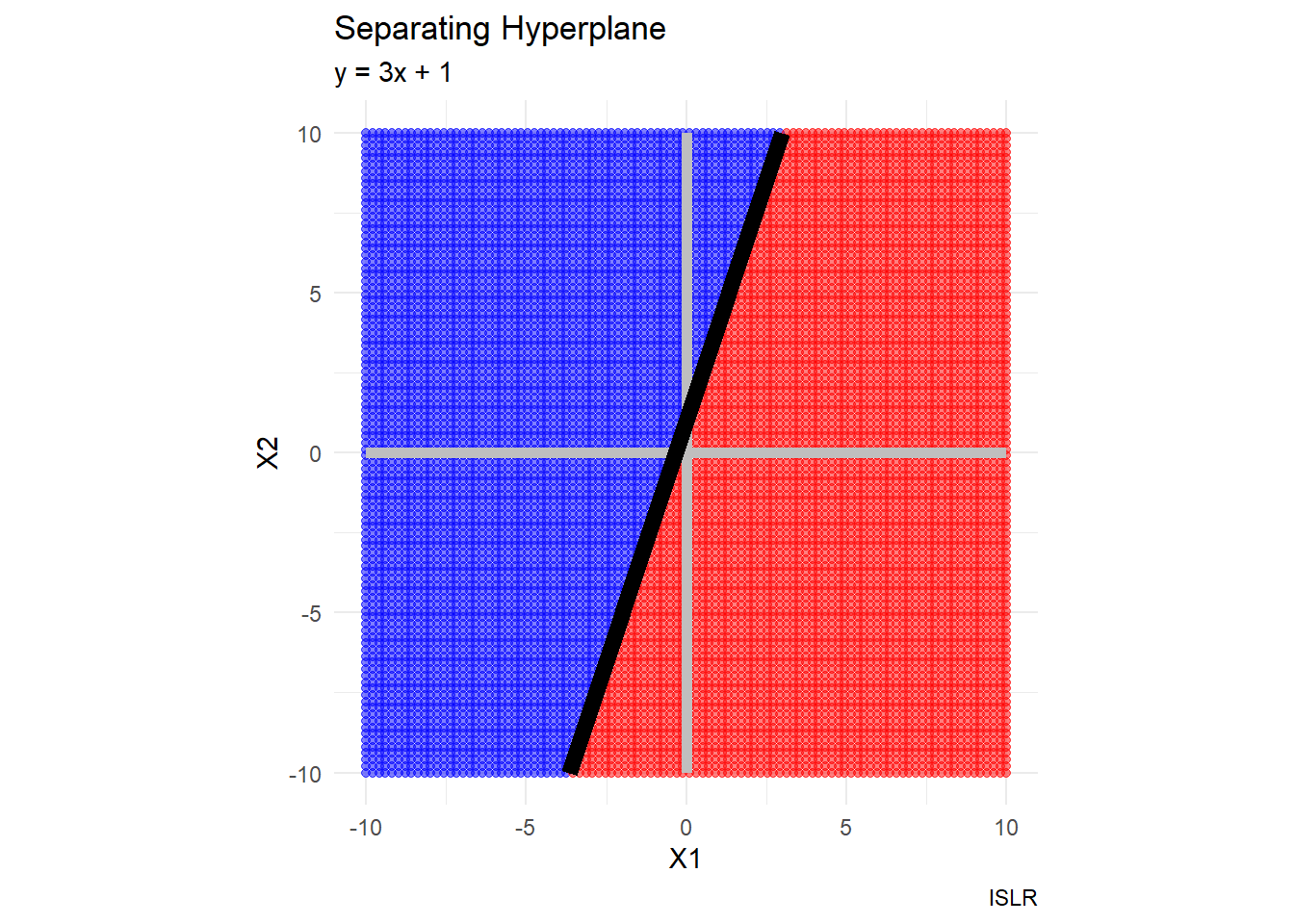``````df1b <- df |>
# math function
mutate(shade = ifelse(yval > 1 - 0.5*xval, "blue", "red"))``````
``````df1b |>
ggplot() +

geom_point(aes(x = xval, y = yval, color = shade),
alpha = 0.5) +
scale_color_identity() +

# axes
geom_segment(aes(x = -10, y = 0, xend = 10, yend = 0),
alpha = 0.25, color = "gray75", linewidth = 2) +
geom_segment(aes(x = 0, y = -10, xend = 0, yend = 10),
alpha = 0.25, color = "gray75", linewidth = 2) +

# main line
geom_segment(aes(x = -10, y = 6, xend = 10, yend = -4),
color = "black", linewidth = 3) +

# customization
coord_equal() +
labs(title = "Separating Hyperplane",
subtitle = "y = 1 - 0.5x",
caption = "ISLR",
x = "X1", y = "X2") +
theme_minimal()``````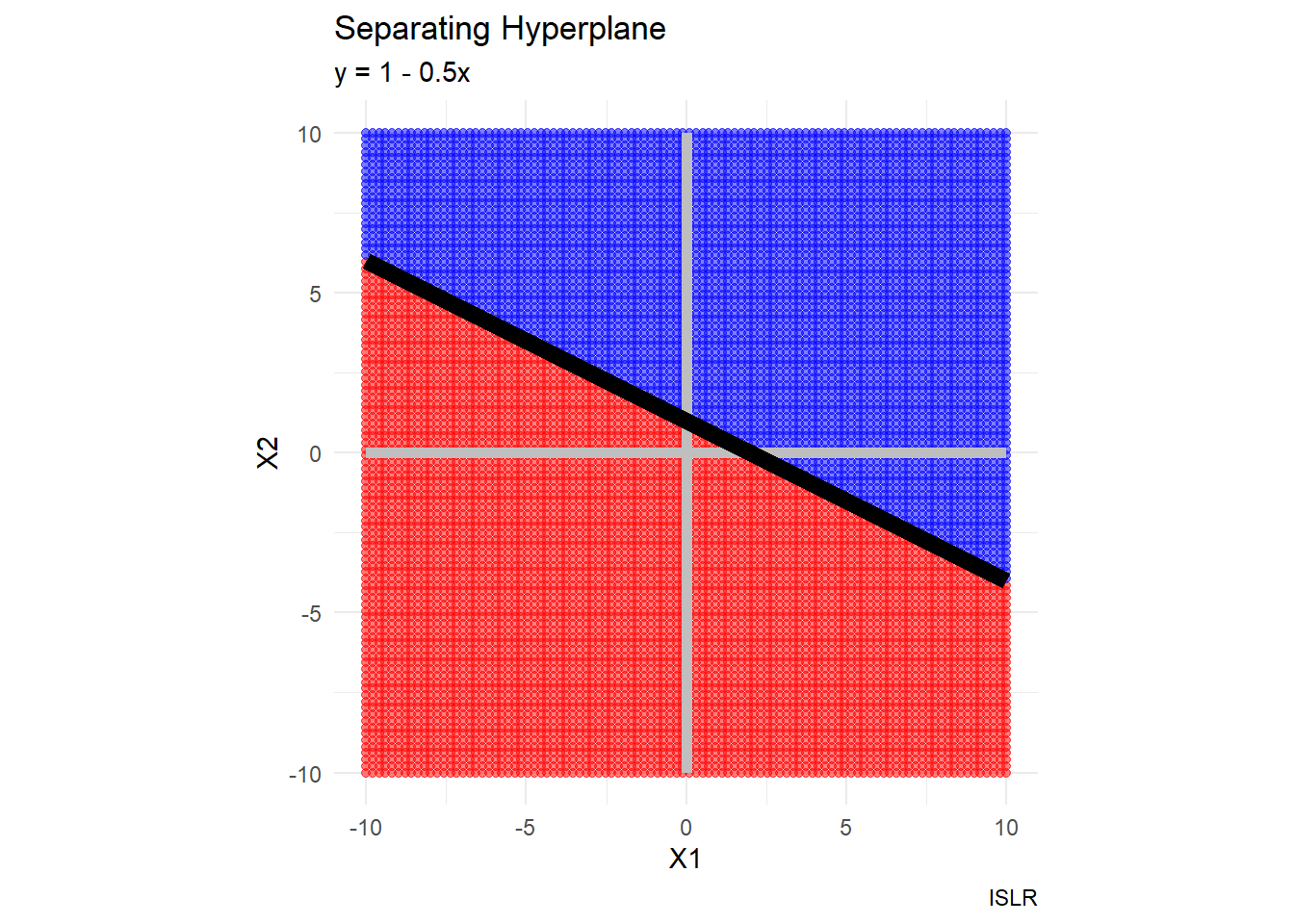``````euclidean_distance <- function(x1, y1, x2, y2){
# computes the Euclidean distance between (x1, y1) and (x2, y2)
sqrt( (x2 - x1)^2 + (y2 - y1)^2 )
}``````
``````df2 <- df |>
# math function
mutate(shade = ifelse(euclidean_distance(xval, yval, -1, 2) > 4,
"blue", "red"))``````
``````df2 |>
ggplot() +

geom_point(aes(x = xval, y = yval, color = shade),
alpha = 0.5) +
scale_color_identity() +

# axes
geom_segment(aes(x = -10, y = 0, xend = 10, yend = 0),
alpha = 0.25, color = "gray75", linewidth = 2) +
geom_segment(aes(x = 0, y = -10, xend = 0, yend = 10),
alpha = 0.25, color = "gray75", linewidth = 2) +

# customization
coord_equal() +
labs(title = "Separating Hyperplane",
subtitle = "(x+1)^2 + (y-2)^2 = 4",
caption = "ISLR",
x = "X1", y = "X2") +
theme_minimal()``````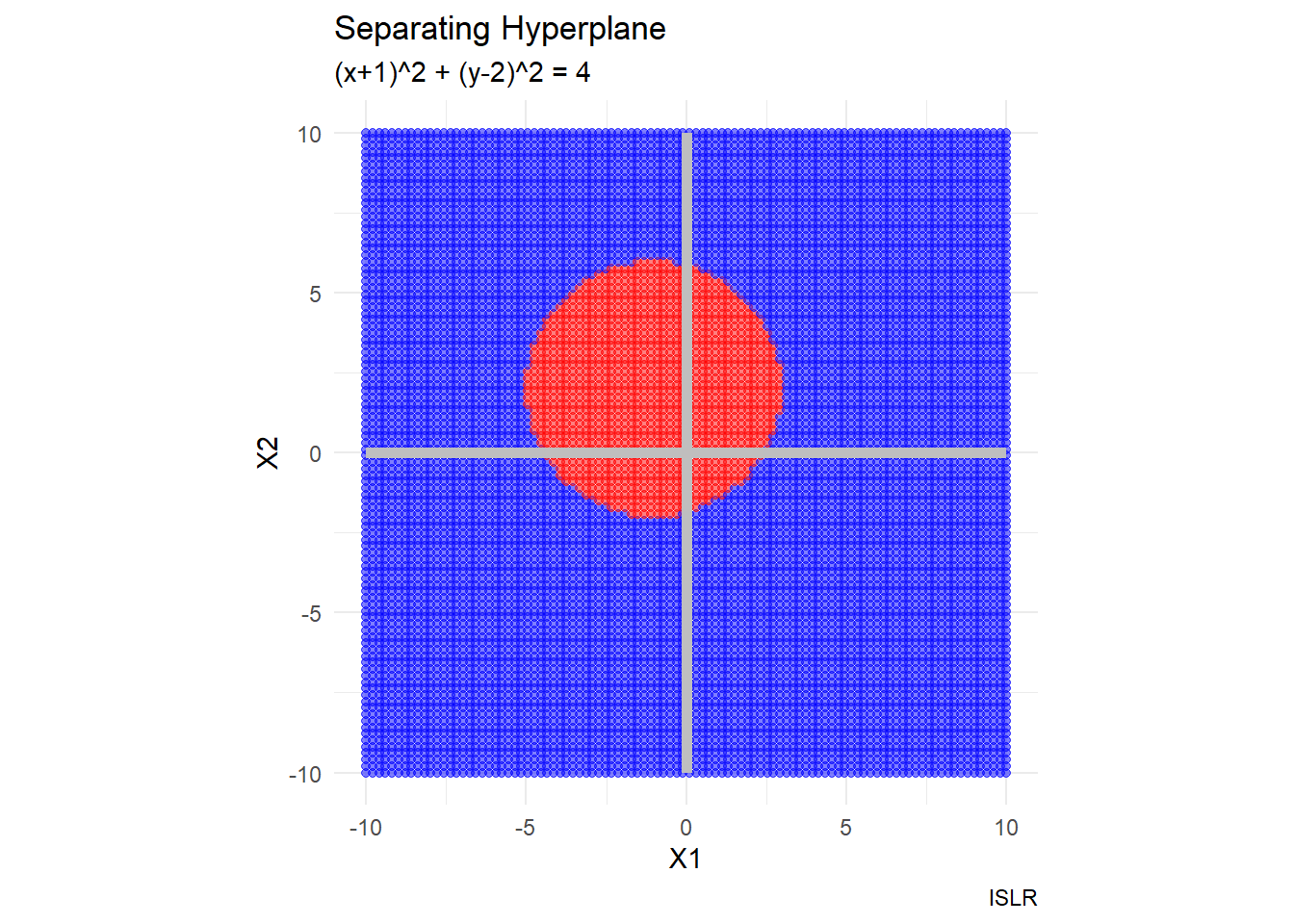``ifelse(euclidean_distance(0, 0, -1, 2) > 4, "blue", "red")``
`` "red"``
``ifelse(euclidean_distance(-1, 1, -1, 2) > 4, "blue", "red")``
`` "red"``
``ifelse(euclidean_distance(2, 2, -1, 2) > 4, "blue", "red")``
`` "red"``
``ifelse(euclidean_distance(3, 8, -1, 2) > 4, "blue", "red")``
`` "blue"``

``````obs <- 1:7
xvals <- c(3,2,4,1,2,4,4)
yvals <- c(4,2,4,4,1,3,1)
class_label <- c("Red", "Red", "Red", "Red", "Blue", "Blue", "Blue")
df3 <- data.frame(obs, xvals, yvals, class_label)``````
``````df3 |>
ggplot() +
geom_point(aes(x = xvals, y = yvals, color = class_label),
size = 5) +
coord_equal() +
scale_color_identity() +
labs(title = "Where to Draw the Separating Hyperplane?",
# subtitle = "y = x - 0.5",
caption = "ISLR",
x = "X1", y = "X2") +
theme_minimal() +
xlim(0,5) + ylim(0,5)``````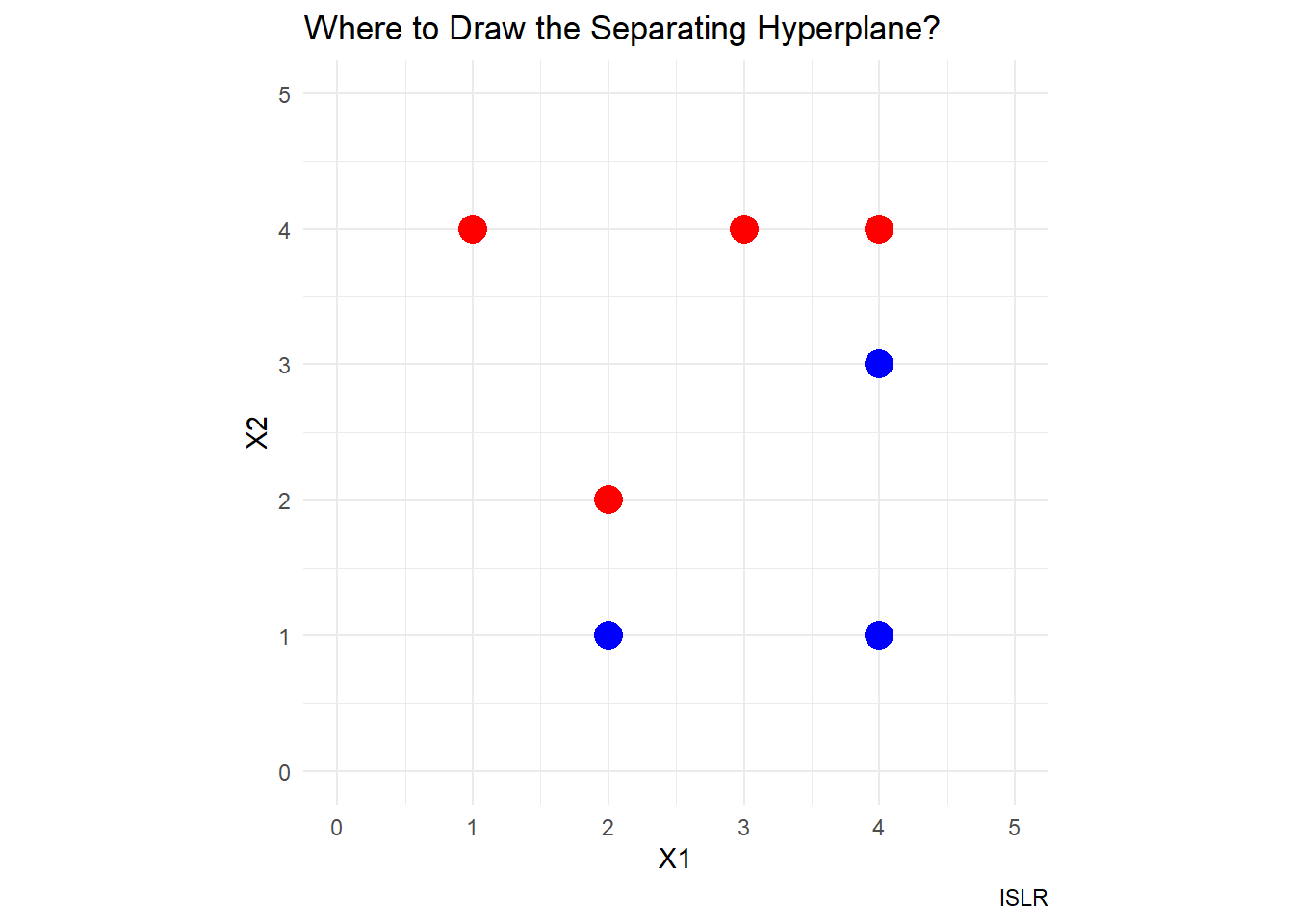``````df3 |>
ggplot() +

# separating hyperplane
geom_segment(aes(x = 0.5, y = 0, xend = 5, yend = 4.5),
color = "black", linetype = 1, linewidth = 3) +

# margins
geom_segment(aes(x = 0, y = 0, xend = 5, yend = 5),
color = "black", linetype = 2, linewidth = 2) +
geom_segment(aes(x = 1, y = 0, xend = 5, yend = 4),
color = "black", linetype = 2, linewidth = 2) +

geom_point(aes(x = xvals, y = yvals, color = class_label),
size = 5) +
coord_equal() +
scale_color_identity() +
labs(title = "Separating Hyperplane",
subtitle = "y = x - 0.5",
caption = "ISLR",
x = "X1", y = "X2") +
theme_minimal() +
xlim(0,5) + ylim(0,5)``````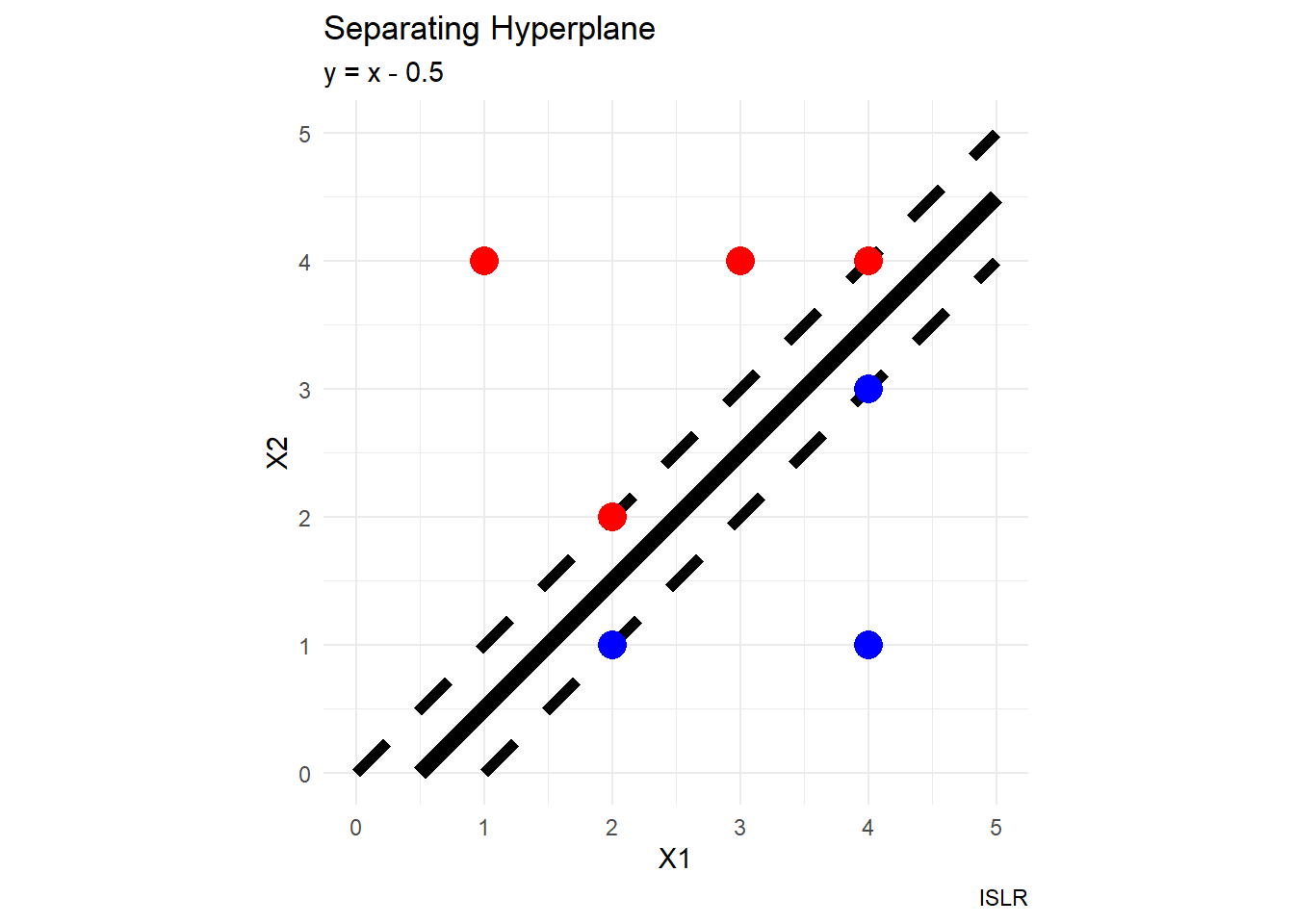``````df3e <- df3 |>
mutate(supp_vec = ifelse(obs %in% c(2,3,5,6),
"support vector", "other data"))

df3e\$supp_vec <- factor(df3e\$supp_vec,
levels = c("support vector", "other data"))

df3e |>
ggplot() +

# separating hyperplane
geom_segment(aes(x = 0.5, y = 0, xend = 5, yend = 4.5),
color = "black", linetype = 1, linewidth = 3) +

# margins
geom_segment(aes(x = 0, y = 0, xend = 5, yend = 5),
color = "black", linetype = 2, linewidth = 2) +
geom_segment(aes(x = 1, y = 0, xend = 5, yend = 4),
color = "black", linetype = 2, linewidth = 2) +

geom_point(aes(x = xvals, y = yvals, color = supp_vec),
size = 5) +
coord_equal() +
scale_color_manual(values = c("purple", "gray50")) +
labs(title = "Separating Hyperplane",
subtitle = "y = x - 0.5",
caption = "ISLR",
x = "X1", y = "X2") +
theme_minimal() +
xlim(0,5) + ylim(0,5)``````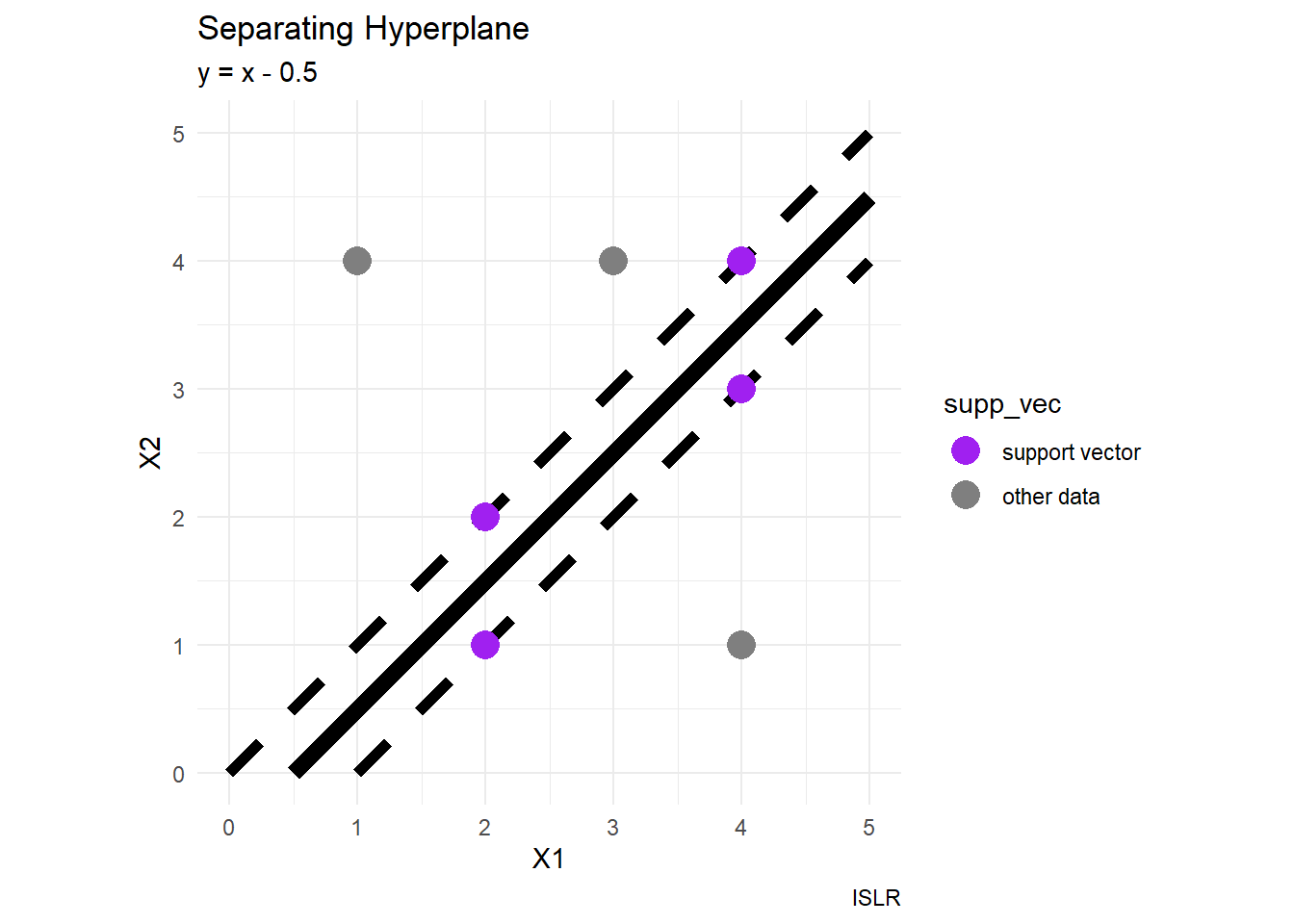``````df3 |>
ggplot() +

# separating hyperplane
geom_segment(aes(x = 0, y = 1/4, xend = 5, yend = 16/4),
color = "black", linetype = 1, linewidth = 3) +

geom_point(aes(x = xvals, y = yvals, color = class_label),
size = 5) +
coord_equal() +
scale_color_identity() +
labs(title = "Separating Hyperplane (not optimal)",
subtitle = "y = 0.25(3x+1)",
caption = "ISLR",
x = "X1", y = "X2") +
theme_minimal() +
xlim(0,5) + ylim(0,5)``````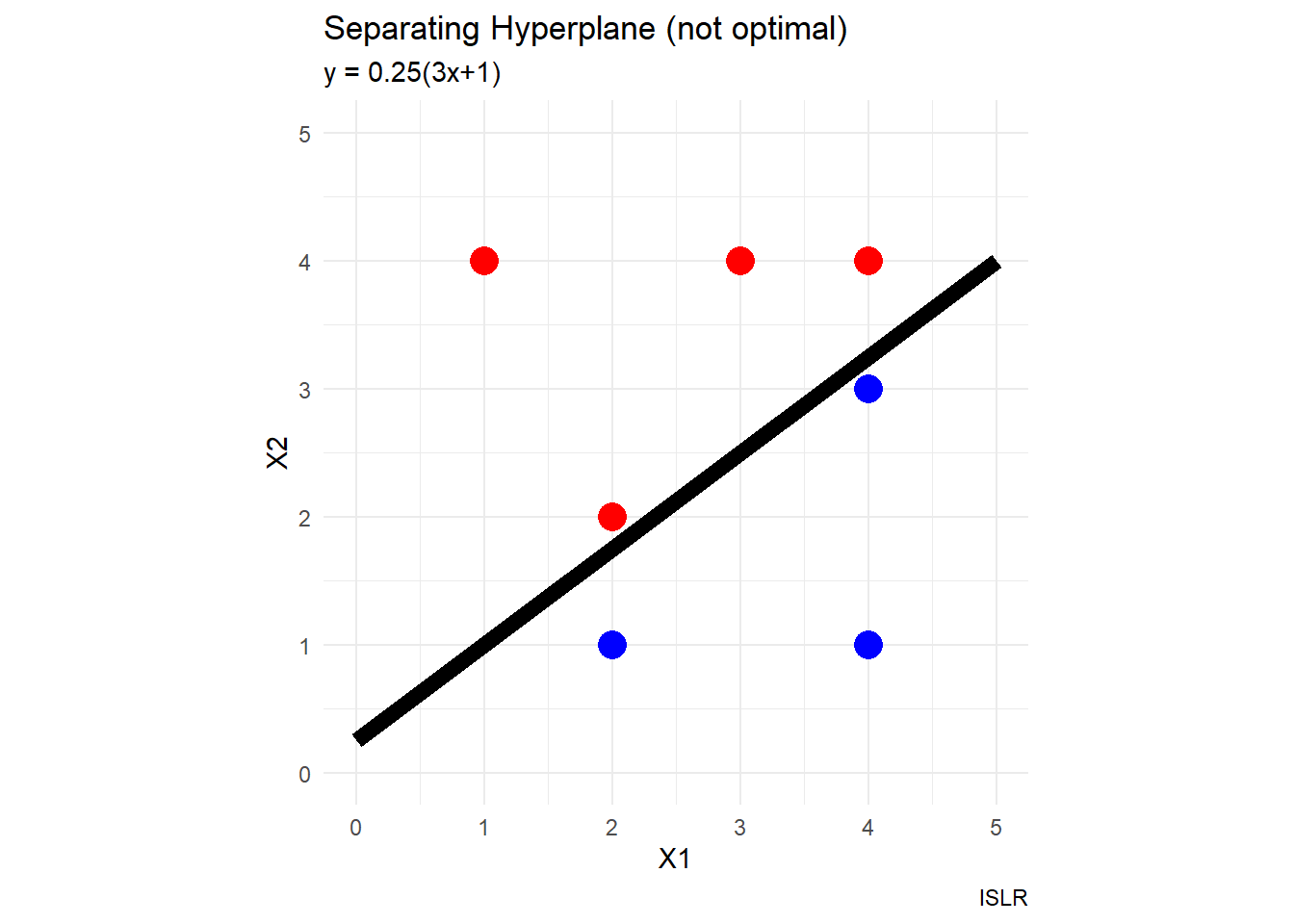``````new_dot <- data.frame(obs = 8, xvals = 0, yvals = 5, class_label = "blue")
df3h <- rbind(df3, new_dot)
df3h |>
ggplot() +
geom_point(aes(x = xvals, y = yvals, color = class_label),
size = 5) +
coord_equal() +
scale_color_identity() +
labs(title = "Where to Draw the Separating Hyperplane?",
subtitle = "new data at (0,5)",
caption = "ISLR",
x = "X1", y = "X2") +
theme_minimal() +
xlim(0,5) + ylim(0,5)``````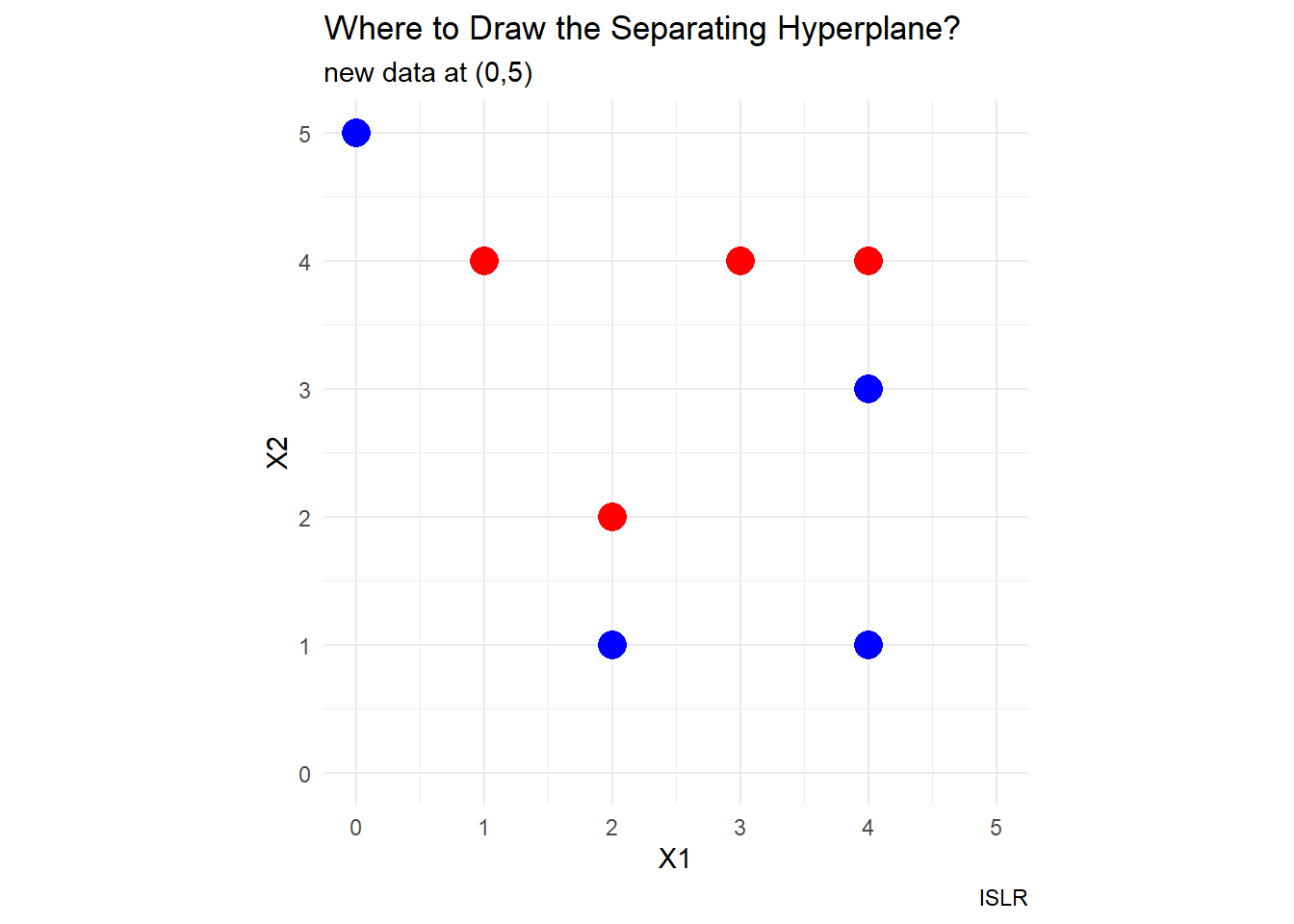``sessionInfo()``
``````R version 4.3.0 (2023-04-21 ucrt)
Platform: x86_64-w64-mingw32/x64 (64-bit)
Running under: Windows 10 x64 (build 19045)

Matrix products: default

locale:
 LC_COLLATE=English_United States.utf8
 LC_CTYPE=English_United States.utf8
 LC_MONETARY=English_United States.utf8
 LC_NUMERIC=C
 LC_TIME=English_United States.utf8

time zone: America/Los_Angeles
tzcode source: internal

attached base packages:
 stats     graphics  grDevices utils     datasets  methods   base

other attached packages:
 ggplot2_3.4.2 dplyr_1.1.2

loaded via a namespace (and not attached):
 vctrs_0.6.2       cli_3.6.1         knitr_1.43        rlang_1.1.1
 xfun_0.39         generics_0.1.3    jsonlite_1.8.4    labeling_0.4.2
 glue_1.6.2        colorspace_2.1-0  htmltools_0.5.5   scales_1.2.1
 fansi_1.0.4       rmarkdown_2.22    grid_4.3.0        munsell_0.5.0
 evaluate_0.21     tibble_3.2.1      fastmap_1.1.1     yaml_2.3.7
 lifecycle_1.0.3   compiler_4.3.0    htmlwidgets_1.6.2 pkgconfig_2.0.3
 rstudioapi_0.14   farver_2.1.1      digest_0.6.31     R6_2.5.1
 tidyselect_1.2.0  utf8_1.2.3        pillar_1.9.0      magrittr_2.0.3
 withr_2.5.0       tools_4.3.0       gtable_0.3.3     ``````